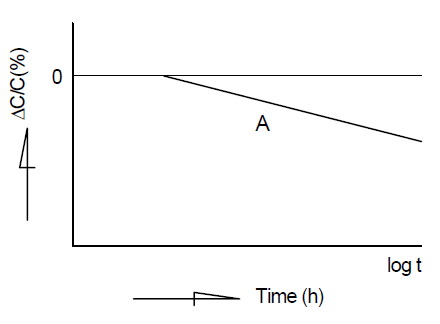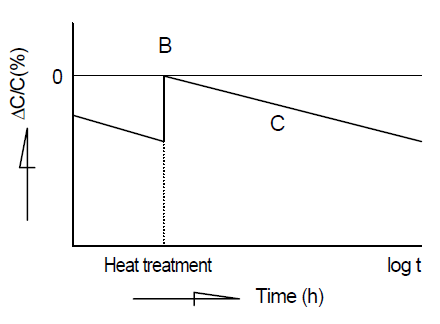# Capacitance aging

Safety Application Guide for Multilayer Ceramic Chip Capacitors
Design
Electrical factors
Capacitance aging

#### The capacitor has the characteristics of which the capacitance decreases according to the passage of time. Since these capacitors may be unable to be used for the time constant circuit etc., for that case, please consult with us.

In ceramic dielectrics of high dielectric constant, capacitance tends to decrease almost linearly to logarithmic time, when the capacitor is left at room temperature without impressed voltage.

Most ceramic dielectrics used for ceramic capacitors have ferroelectric characteristics, and exhibit a curie temperature. Above this temperature, the dielectrics have a highly symmetric cubic crystal structure whereas below the curie temperature, the crystal structure is less symmetrical. Although in single crystals this phase transition is very sharp, in practical ceramics it is often spread over a finite temperature range. In all cases it is linked with a peak in the capacitance/temperature curve.
Under the influence of thermal vibration, the ions in the crystal lattice continue to move to positions of lower potential energy for a long time after the dielectric has cooled down below the curie temperature. This makes capacitance aging, whereby a capacitor's capacitance continually decreases. (Line A in the below graph) However, if the capacitor is heated to a temperature above the curie temperature, de-aging takes place and the capacitance lost through aging is regained. (B point in the below graph) The aging recommences when the capacitor cools down below its curie temperature. (Line C in the below graph)

This is a phenomenon of shifting to a lower energy state by which the ceramic dielectric becomes more stable. Therefore, take capacitance aging into consideration when using a capacitor with Class 2 or Class 3 ceramic dielectrics for a circuit with a narrow range of allowable capacitance change, such as a time constant circuit.
Since the effects of this aging can be reversed, a dielectric's capacitance can be returned to its original value by subjecting it to a higher temperature than its Curie point, e.g. 125°C for BaTiO3. The phenomena can been noticed immediately after soldering or after reworking/repair with a soldering iron. The examples of capacitance aging are shown as follows.

Capacitance aging at room temperature
Capacitance aging of before-and-after heat treatment